# Закажи себе WolframAlpha|Pro! Получи пошаговые решения твоих задач!

## ЕГЭ с Wolfram|Alpha :: 08.05.14 :: Резерв 1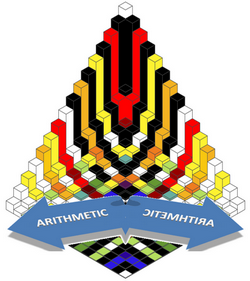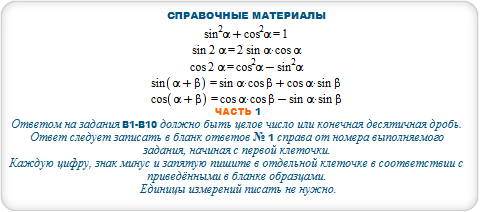#### ceil(a/(b-c)) for a = 3100, b = 900, c=300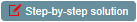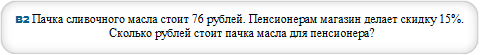#### 85% of 76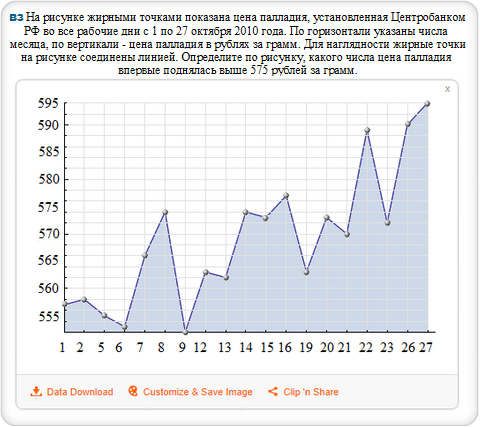#### plot(557,558,555,553,566,574,552,563,562,574,573,577,563,573,570,589,572,590,594)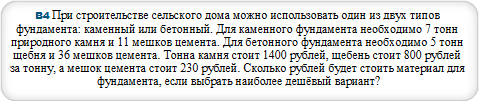#### B=m*v+n*w where m=5,n=36,v=800,w=230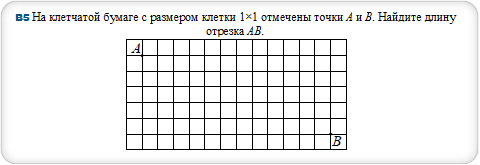#### triangle (1,1),(1,6),(13,1)#### 9/45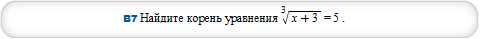#### solve (x+3)^(1/3)=5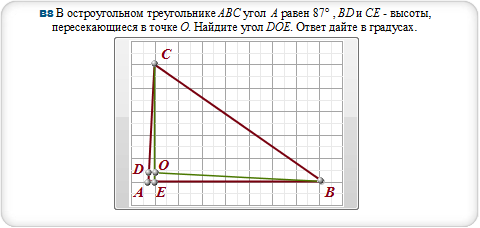#### polygon [16.3,2],[1.47,2],[2,12],[16.3,2],[1.5, 2.8],[1.47,2],[2,2],[2,12]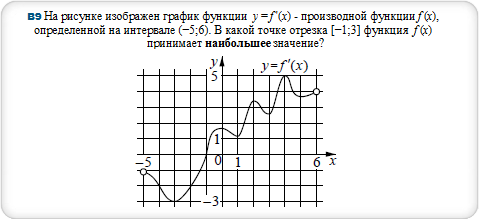#### extrema calculator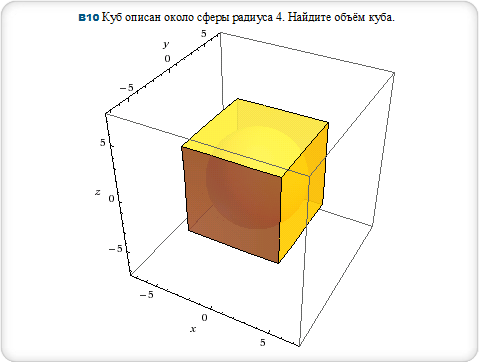#### sphere radius 4, cube edge length 8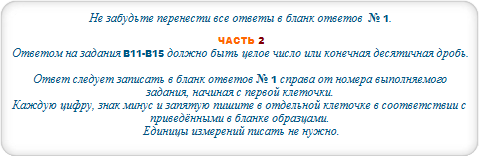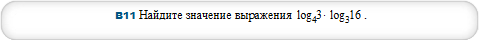#### log(4,3)*log(3,16)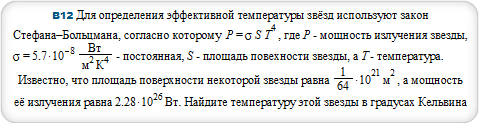#### ((2.28*10^26*64)/(5.7*10^(-8)*10^21))^(1/4)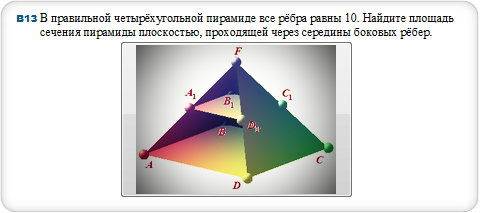#### square pyramid with height 5*sqrt2, base 10 and square pyramid with height 5*sqrt2/2, base 5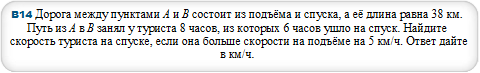#### v = (a*(T-t)+s)/T where s=38,T=8,t=6,a=5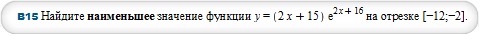#### minimize (2*x+15)*E^(2*x+16) for x=-12..-2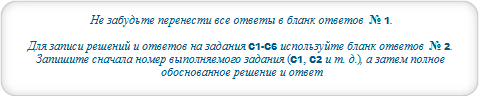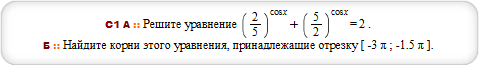#### plot ((2/5)^cos(x),2-(5/2)^cos(x)) for x from-3*Pi to -3*Pi/2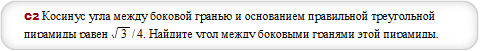#### triangular pyramid with height sqrt13/5, base 1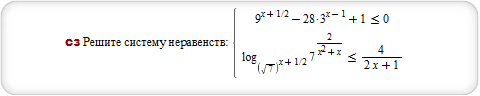#### plot (9^(x+1/2)-28*3^(x-1)+1, log(sqrt(7)^(x+1/2),7^(2/(x^2+x)))-4/(2*x+1)),x=-4..4,y=-8..4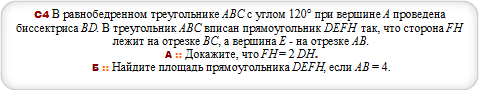#### triangle [0,2],[3.5,0],[-3.5,0], polygon [-1.3,1.3],[1.3,1.3], [1.3,0],[-1.3,0]#### plot (4*(3.5*x-2.5)^(1/3)+3*log(2,3*x-1),-4*(-3.5*x+2.5)^(1/3)+3*log(2,3*x-1)), x=1/3..2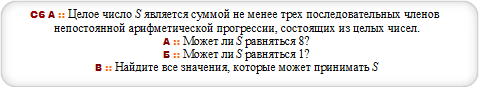#### -1,0,1,2,3,4,...